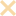Or

##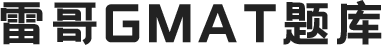• 2018年6月出版，OG19新题即OG2019综合册里与OG18相比新增的题目。此内容做为官方题源可以帮助大家更好的需要了解考点，了解考试趋势和考官思维。OG建议大家刷3遍，第一遍刷纸质，第二遍计时精刷，第三遍刷错题。做完后记得及时分析总结。

142
题目总数

16116
做题总人数

78.6%
平均准确率##### 热门讨论
• 【数据充分DS】

The cost of each adult's ticket for a certain concert was \$30, and the cost of each child's ticket for the concert was \$24. If Hannah purchased tickets for this concert, what was the average (arithmetic mean) cost per ticket?(1) Hannah purchased twice as many children's tickets as adults' tickets.(2) Hannah purchased 4 children's tickets.

• 【数据充分DS】

If the prime numbers p and t are the only prime factors of the integer m, is m a multiple of p2  t ?(1) m has more than 9 positive factors.(2) m is a multiple of p3 .

• 【数据充分DS】

If m and r are two numbers on a number line, what is the value of r ?(1) The distance between r and 0 is 3 times the distance between m and 0 .(2) 12 is halfway between m and r.

• 【数据充分DS】

If t is a positive integer and r is the remainder when t2  + 5t + 6 is divided by 7, what is the value of r ?(1) When t is divided by 7, the remainder is 6.(2) When t2 is divided by 7, the remainder is 1.

• 【数据充分DS】

If n is a positive integer and r is the remainder when n2 - 1 is divided by 8, what is the value of r ?(1) n is odd.(2) n is not divisible by 8.

• 【数据充分DS】

If n is an integer between 2 and 100 and if n is also the square of an integer, what is the value of n ?(1) n is even.(2) The cube root of n is an integer.

• 【数据充分DS】

If the product of the three digits of the positive integer k is 14, what is the value of k ? (1) k is an odd integer.  (2) k < 700

• 【数据充分DS】

If \$1,000 is deposited in a certain bank account and remains in the account along with any accumulated interest, the dollar amount of interest I , earned by the deposit in the first n years is given by the formula I =  , where r percent is the annual interest rate paid by the bank. Is the annual interest rate paid by the bank greater than 8 percent? (1) The deposit earns a total of \$210 in interest in the first two years. (2)

• 【数据充分DS】

Each of the students in a certain class received a single grade of P, F, or I. What percent of the students in the class were females? (1) Of those who received a P, 40 percent were females. (2) Of those who received either an F or I, 80 percent were males.

• 【数据充分DS】

If -2x > 3y, is x negative?(1) y > 0(2) 2x + 5y - 20 = 0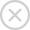• 手机注册
• 邮箱注册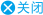###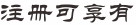•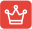#### 知识库学习

GMAT语法、逻辑、阅读、数学各单项备考知识点学习及测验
•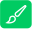#### 在线做题

包含GMAT各单项必考知识点题目、OG/PREP/GWD/雷哥讲义题目、难度650/680/700/730题库题目练习及题目解析
•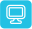#### 在线模考

语文套题/数学套题/全套仿真模考，包含GWD/PERP/精选模考等上百套套题模考
•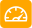#### 在线测评

适合5种不同基础的GMAT学员，测评后可自动出具分数报告及复习计划指导
•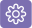#### 资料下载

GMAT必备备考资料下载、鸡精下载、课程课件等免费下载
•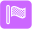#### 课程学习

注册会员后，可在GMAT课程区，选择免费直播课程及公开课程进行在线学习
×